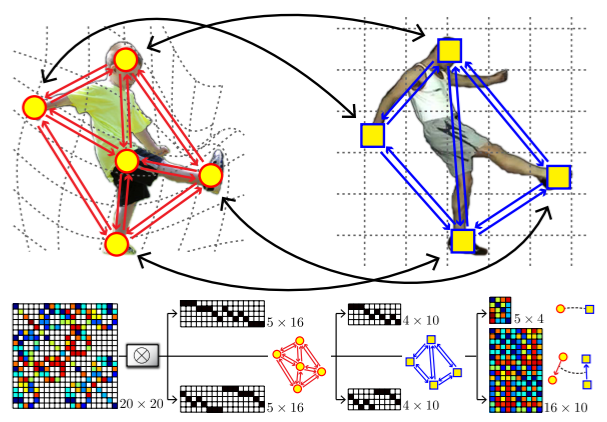# src.factorize_graph_matching.construct_sparse_aff_mat¶

src.factorize_graph_matching.construct_sparse_aff_mat(Ke: torch.Tensor, Kp: torch.Tensor, row_idx: torch.Tensor, col_idx: torch.Tensor)[source]

Construct sparse affinity matrix with edge-wise affinity matrix $$\mathbf{K}_e$$, node-wise matrix $$\mathbf{K}_p$$ and graph connectivity matrices $$\mathbf{G}_1, \mathbf{H}_1, \mathbf{G}_2, \mathbf{H}_2$$

$\mathbf{K}=\mathrm{diag}(\mathrm{vec}(\mathbf{K}_p)) + (\mathbf{G}_2 \otimes_{\mathcal{K}} \mathbf{G}_1) \mathrm{diag}(\mathrm{vec}(\mathbf{K}_e)) (\mathbf{H}_2 \otimes_{\mathcal{K}} \mathbf{H}_1)^\top$

where $$\mathrm{diag}(\cdot)$$ means building a diagonal matrix based on the given vector, and $$\mathrm{vec}(\cdot)$$ means column-wise vectorization. $$\otimes_{\mathcal{K}}$$ denotes Kronecker product.

This function does not supports batched operations. This formulation is developed by “F. Zhou and F. Torre. Factorized Graph Matching. TPAMI 2015.”

Parameters
• Ke$$(1\times n_e)$$ non-zero elements in edge-wise affinity matrix Ke. $$n_e$$: number of non-zero elements in dense Ke

• Kp$$(1\times n_1 n_2)$$ non-zero elements in node-wise affinity matrix Kp. $$n_1$$: number of nodes in graph 1, $$n_2$$: number of nodes in graph 2

• row_idx$$(1\times n_e)$$ row indices of the non-zero elements in edge-wise affinity matrix Ke

• col_idx$$(1\times n_e)$$ column indices of the non-zero elements in edge-wise affinity matrix Ke

Returns

none-zero values in the affinity matrix $$\mathbf K$$, and their row/column indices (value, row-idx, col-idx)

For a graph matching problem with 5 nodes and 4 nodes, the connection of $$\mathbf K$$ and $$\mathbf{K}_p, \mathbf{K}_e$$ is illustrated aswhere $$\mathbf K (20 \times 20)$$ is the complete affinity matrix, $$\mathbf{K}_p (5 \times 4)$$ is the node-wise affinity matrix, $$\mathbf{K}_e(16 \times 10)$$ is the edge-wise affinity matrix.